# Introduction to Network Mathematics

By Bruce Hoppe

 If your browser does not support mathematical symbols, you will not see this document correctly. If "⊆" looks like a box in quotes instead of a lazy U, or this math-symbol-test-page looks like a bunch of boxes, then you need to find a browser that supports mathematical symbols. In our experience, Firefox works and Internet Explorer does not.

Introduction to Network Mathematics provides college students with basic graph theory to better understand the Internet. Many passages are edited from Wikipedia, a few are from PlanetMath, and others are original writing by Bruce Hoppe, who teaches CS-103: "Introduction to Internet Technologies and Web Programming" at Boston University. This is a work in progress, first created in the spring semester of 2007, and now being used in the fall semester 2008.

Copyright © 2007, 2008 by Bruce Hoppe. Permission is granted to copy, distribute and/or modify these documents under the terms of the GNU Free Documentation License, Version 1.2 or any later version published by the Free Software Foundation; with no Invariant Sections, with no Front-Cover Texts, and with no Back-Cover Texts. A copy of the license is included in the section entitled "GNU Free Documentation License". If you believe a portion of this site infringes on your or anyone's copyright, please send us a note.

# Sets

A set is a collection of objects. These objects are called the elements or members of the set. Objects can be anything: numbers, people, other sets, etc. For instance, 4 is a member of the set of all even integers. Clearly, the set of even integers is infinitely large; there is no requirement that a set be finite.

If x is a member of A, then we write xA. We may also say that x "belongs to" A, or that x "is in" A.

If x is not a member of A, then we write xA.

Two sets A and B are defined to be equal when they have precisely the same elements, that is, if every element of A is an element of B and every element of B is an element of A. Thus a set is completely determined by its elements; the description is immaterial. For example, the set with elements 2, 3, and 5 is equal to the set of all prime numbers less than 6.

If the sets A and B are equal, then we write A = B.

We also allow for an empty set, a set without any members at all. We write the empty set as { }.

## Explicit and Implicit Set Notation

Explicit Set Notation: The simplest way to describe a set is to list its elements between curly braces, known as defining a set explicitly. Thus {1,2} denotes the set whose only elements are 1 and 2. Note the following points:

• Order of elements is immaterial: for example, {1,2} = {2,1}.
• Repetition of elements is irrelevant: for example, {1,2,2} = {1,1,1,2} = {1,2}.

Warning: This notation can be informally abused by saying something like {dogs} to indicate the set of all dogs, but this example would be read by mathematicians as "the set containing the single element dogs".

The simplest possible example of explicit set notation is { }, which denotes the empty set.

Implicit Set Notation: We can also use the notation {x : P(x)} to denote the set containing all objects for which the condition P holds, known as defining a set implicitly. For example, {x : x is a real number} denotes the set of real numbers, {x : x has blonde hair} denotes the set of everything with blonde hair, and {x : x is a dog} denotes the set of all dogs.

## Cardinality

Given a finite set A, the cardinality of A is simply the number of elements in A. We write the cardinality of A as |A|, which we may read as "cardinality of A" or "size of A." Note that this vertical-bar notation is the same as used to denote absolute value, but the meaning of cardinality is quite different from absolute value. In particular, absolute value operates on numbers (e.g., |-3| = 3) while cardinality operates on sets (e.g., |{-3}| = 1). Other examples of cardinality are:
• |{3,4}| = 2.
• |{ }| = 0. The empty set has no elements.
• |{{1,2},{3,4}}| = 2. In this case the two elements of {{1,2},{3,4}} are themselves sets: {1,2} and {3,4}.
• |{x: x is registered for CS-103}| = the number of people registered for CS-103.

We can also consider the cardinality of an infinite set A, but that discussion is beyond the scope of this introduction.

## Subsets

Given two sets A and B we say that A is a subset of B if every element of A is also an element of B. Notice that in particular, B is a subset of itself; a subset of B that isn't equal to B is called a proper subset.

If A is a subset of B, then one can also say that B is a superset of A, that A is contained in B, or that B contains A. In symbols, AB means that A is a subset of B, and BA means that B is a superset of A. For clarity, we use "⊆" and "⊇" for subsets (which allow set equality) and we use "⊂" and "⊃" are reserved for proper subsets (which exclude equality).

For example, let A be the set of American citizens, B be the set of registered students at Boston University, and C be the set of registered students in CS-103. Then C is a proper subset of B and B contains C, which we can write as C ⊂ B andBC. If we allow the possibility that every BU student might be registered for CS-103, then we would write C ⊆ B or BC to allow for this unlikely situation. Note that not all sets are comparable in this way. For example, it is not the case either that A is a subset of B nor that B is a subset of A.

## Unions, intersections, and relative complements

Given two sets A and B, the union of A and B is the set consisting of all objects which are elements of A or of B or of both. It is written as AB.

The intersection of A and B is the set of all objects which are both in A and in B. It is written as AB.

Finally, the relative complement of B relative to A, also known as the set theoretic difference of A and B, is the set of all objects that belong to A but not to B. It is written as A \ B. Symbolically, these are respectively

A ∪ B := {x : (xA) or (xB)}
AB := {x : (xA) and (xB)}
A \ B := {x : (xA) and not (xB) }

Notice that B doesn't have to be a subset of A for A \ B to make sense.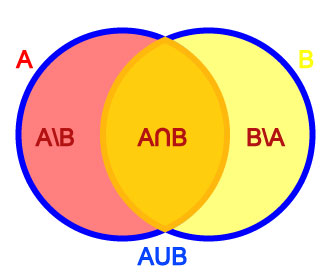To illustrate these ideas, let A be the set of left-handed people, and let B be the set of people with blond hair. Then AB is the set of all left-handed blond-haired people, while AB is the set of all people who are left-handed or blond-haired or both. A \ B, on the other hand, is the set of all people that are left-handed but not blond-haired, while B \ A is the set of all people that have blond hair but aren't left-handed.

Venn diagrams are illustrations that show these relationships pictorally. The above example can be drawn as the Venn diagram at right:

Disjoint sets: Let E be the set of all human beings, and let F be the set of all living things over 1000 years old. What is EF in this case? No human being is over 1000 years old, so EF must be the empty set { }. When the intersection of any two sets is empty, we say those two sets are disjoint.

## Ordered Pairs and Ordered Lists

Intuitively, an ordered pair is simply a collection of two objects such that one can be distinguished as the first element and the other as the second element, and having the fundamental property that two ordered pairs are equal if and only if their first elements are equal and their second elements are equal.

We write an ordered pair using parentheses--not curly braces, which are used for sets. For example, the ordered pair (a,b) consists of first element a and second element b.

Two ordered pairs (a,b) and (c,d) are equal if and only if a = c and b = d.

In contrast, a set of two elements is an unordered pair which we write using curly braces and where there is no distinction between the first element and the second element. It follows that
• Two ordered pairs (a,b) and (c,d) are equal if and only if a = c and b = d
• Two sets {a,b} and {c,d} are equal if and only if
1. Either a = c and b = d
2. Or a = d and b = c
We can also write ordered lists having any finite number of elements with an intuitive extension of the above notation. For example:
• (1,2,3) is an ordered list. Note that (1,2,3) is different from (3,2,1).
• {1,2,3} is a set. Note that {1,2,3} = {3,2,1}.
• {(1,2),(3,4)} is a set of two elements, each of which is an ordered pair. One of the elements of the set is (1,2) and the other element of the set is (3,4).
• ({1,2},{3},{2,4}) is an ordered list of sets. The first element of the list is the set {1,2}; the second element of the list is the set {3}; the third element of the list is the set {2,4}.

## Partitions

A partition of a set X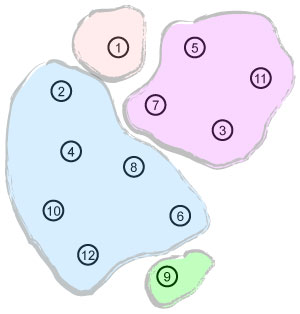is a division of X into non-overlapping subsets such that every element of X is in exactly one of these subsets.

Equivalently, a set P of subsets of X, is a partition of X if

1. The union of the elements of P is equal to X.
2. The intersection of any two elements of P is empty.

We also typically require that each subset of a partition be non-empty. For example:

• The Venn diagram at right represents one possible partition of the set of all integers from 1 to 12.
• If A is the set of all integers, E is the set of even integers, and O is the set of odd integers, then {E,O} is a parition of A.
• If B is the set of all registered BU students and C is the set of all registered CS-103 students, then {C,B\C} is a partition of B.
• For any set X, P = {X} is a partition of X.
• Every singleton set {x} has exactly one partition, namely { {x} }.
• The set { 1, 2, 3 } has these five partitions:
1. { {1}, {2}, {3} }
2. { {1, 2}, {3} }
3. { {1, 3}, {2} }
4. { {1}, {2, 3} }
5. { {1, 2, 3} }

# Graphs

Mathematicians have developed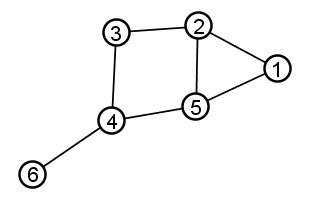graph theory in order to study all kinds of networks. A graph is a set of objects connected by lines.

The objects in a graph are usually called nodes or vertices. The lines connecting the objects are usually called links or edges.

More formally, we define a graph G as an ordered pair G = (V,E) where

• V is a set of vertices (nodes)
• E is a set of edges (links).
• Each edge is a pair of vertices. In other words, each element of E is a pair of elements of V.

Example: The picture above represents the following graph:

• V = {1,2,3,4,5,6}
• E = {{1,2},{1,5},{2,3},{2,5},{3,4},{4,5},{4,6}}
Loops: An edge with identical endpoints is a loop: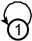Unless explicitly stated otherwise, we disallow loops.

Trivial and empty graphs: The graph with only one vertex and no edges is called the trivial graph. The graph with no vertices and no edges is called the empty graph.

## Undirected and Directed Graphs

Undirected graph: The edges of a graph are assumed to be unordered pairs of vertices. Sometimes we say undirected graph to emphasize this point. In an undirected graph, we write edges using curly braces to denote unordered pairs. For example, an undirected edge {2,3} from vertex 2 to vertex 3 is the same thing as an undirected edge {3,2} from vertex 3 to vertex 2.

Directed graph: In a directed graph, the two directions are counted as being distinct directed edges. In an directed graph, we write edges using parentheses to denote ordered pairs. For example, edge (2,3) is directed from 2 to 3 , which is different than the directed edge (3,2) from 3 to 2. Directed graphs are drawn with arrowheads on the links, as shown below:Two vertices are called adjacent if they share a common edge, in which case the common edge is said to join the two vertices. An edge and a vertex on that edge are called incident.

See the 6-node graph below right for examples of adjacent and incident:

• Nodes 4 and 6 are adjacent (as well as many other pairs of nodes)
• Nodes 1 and 3 are not adjacent (as well as many other pairs of nodes)
• Edge {2,5} is incident to node 2 and node 5.

## Degree

The degree of a vertex is the total number of vertices adjacent to the vertex. The degree of a vertex v is denoted deg(v).The undirected graph at right has the following degrees:

Vertex Degree
1 2
2 3
3 2
4 3
5 3
6 1

In a directed graph, we define degree exactly the same as above (and note that "adjacent" does not imply any direction or lack of direction). It is also important to define indegree and outdegree. Recall that any directed edge has two distinct ends: a head (the end with an arrowhead) and a tail. Each end is counted separately. The sum of head endpoints count toward the indegree of a vertex and the sum of tail endpoints count toward the outdegree of a vertex. The directed graph at right has the following degrees, indegrees, and outdegrees: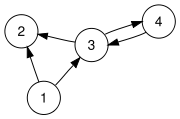Vertex Degree Indegree Outdegree
1 2 0 2
2 2 2 0
3 3 2 2
4 1 1 1

Pay particular attention to nodes 3 and 4 in the above table. If they seem confusing, try re-drawing the above graph using the "easier way to draw" illustrated previously.

## Density and Average Degree

The graph density of a graph G = (V,E) measures how many edges are in set E compared to the maximum possible number of links between vertices in set V. Density is calculated as follows:

• An undirected graph with no loops can have at most |V| * (|V| − 1) / 2 edges, so the density of an undirected graph is 2 * |E| / (|V| * (|V| − 1)).
• A directed graph with no loops can have at most |V| * (|V| − 1) edges, so the density of a directed graph is |E| / (|V| * (|V| − 1))
The average degree of a graph G is another measure of how many edges are in set E compared to number of vertices in set V. Because each edge is incident to two vertices and counts in the degree of both vertices, the average degree of a graph is 2*|E|/|V|

## Neighborhood

The neighborhood of a vertex v in a graph G is the set of vertices adjacent to v. The neighborhood is denoted N(v). The neighborhood does not include v itself. Neighborhoods are also used in the clustering coefficient of a graph, which is a measure of the average density of its neighborhoods.

## Paths and Walks

A path P = (v1,v2,v3,...,vn) is an ordered list of vertices such that

1. From each of its vertices there is an edge to the next vertex in the list
2. No vertex occurs in the list more than once.

We say walk to denote an ordered list of vertices that follows the first requirement above but not the second. (In other words, a walk may visit the same vertex more than once, but a path must never do so.) The first vertex is called the origin and the last vertex is called the destination. Both origin and destination are called endpoints of the path. The other vertices in the path are internal vertices. A cycle is a walk such that the origin and destination are the same; a simple cycle is a cycle that does not repeat any vertices other than the origin and destination.

The same concepts apply both to undirected graphs and directed graphs, with the edges being directed from each vertex to the following one. Often the terms directed path and directed cycle are used in the directed case.

Example: In the graph below, there are many paths from node 6 to node 1. One such path is P1 = (6, 4, 3, 2, 5, 1); another path from 6 to 1 is P2 = (6, 4, 5, 1).## Connected Components

A connected graph is one where each vertex can reach every other vertex by one or more paths. A connected graph is said to consist of a single connected component. If a graph is not connected, then it consists of two or more connected components. Intuitively, connected components are easy to see: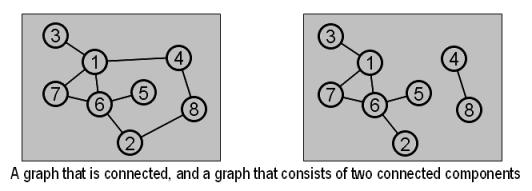More formally, a connected component is a subgraph with the following two properties:

1. Each vertex in the subgraph can reach every other vertex in the subgraph by one or more paths
2. T here is no edge from any vertex inside the subgraph to any vertex outside the subgraph. (This means the subgraph can't get any bigger without losing connectivity; it is maximal.)

## Length, Distance, Diameter

The length of a path or walk is the number of edges that it uses, counting multiple edges multiple times. In the graph below, (5, 2, 1) is a path of length 2 and (1, 2, 5, 1, 2, 3) is a walk of length 5.The distance between two vertices x and y is written d(x,y) and defined as the length of the shortest path from x to y. If there is no path from x to y (i.e., if x and y are in different connected components), then d(x,y) is infinity.

The diameter of a graph G is the maximum distance between any two vertices in G. If G is not connected, then the diameter of G is infinity.

## Cliques and Independent Sets

In a complete graph, every pair of vertices is joined by an edge.Given a graph, a clique is a subset of vertices such that every pair of vertices in the subset is joined by an edge. The size of a clique is the number of vertices it contains. The red nodes {6,8,12} below are a clique of size three: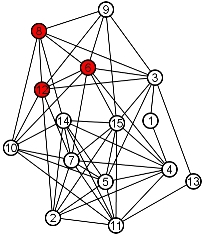It is an interesting and very hard problem to find the largest clique in a graph-- known as the maximum clique problem. Can you find the largest clique in the graph above? Below is an example of an easier version of this problem.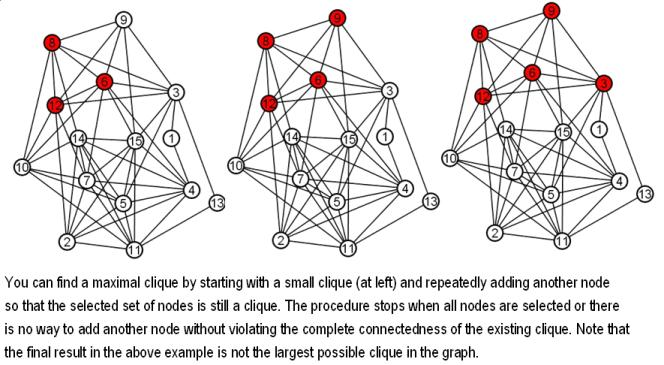The opposite of a clique is an independent set. An independent set is a subset of vertices such that for every two vertices in the subset, there is no edge connecting the two. The size of an independent set is the number of vertices it contains.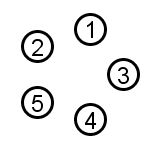The vertices of any edgeless graph make an independent set, rather trivially:

Given an arbitrary graph (with edges), it is an interesting and hard problem to find the largest independent set in the graph. This is known as the maximum independent set problem. The blue nodes below are a maximum independent set:

## Induced Subgraphs

It is often useful to consider only part of a graph. Induced subgraphs are one particularly convenient way to define a specific sub-part of a larger graph.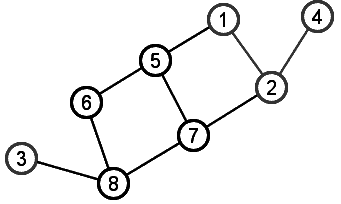Graph G = (V,E)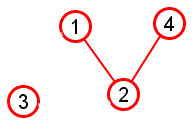Subgraph induced by subset of nodes {1,2,3,4}

Given a graph G=(V,E), an induced subgraph is defined by a subset of vertices S ⊆V. Then the nodes of the subgraph induced by S are simply the nodes in S, and the edges of the subgraph induced by S are all edges with both endpoints in S.

The upper graph pictured at right shows G=(V,E) with V={x: x is an integer from 1 to 8} and edges as drawn.

The lower picture shows the subgraph induced by the subset of nodes S={1, 2, 3, 4}. Notice that this induced subgraph consists of two connected components, even though the graph as a whole is a single connected component.

Another Example: In lecture we have discussed the Facebook friends network. We can write this as a graph using implicit notation as follows: G = (V,E), where V = {x: x is a person on Facebook} and E = {{x,y}: xV and yV and (x and y are friends on Facebook)}. The Facebook friends network is enormous, and so graph G is impossible to draw by hand; however, we can choose a small subset S of nodes in V and draw the graph induced by that subset S. This is exactly what the Touchgraph Facebook browser does: It lets you choose set S to be some number of your "Top Friends" on Facebook, possibly with the addition of yourself. Given this set S, Touchgraph displays a subgraph of the entire Facebook friends network: specifically, the subgraph induced by your chosen number of "Top Friends" (possibly with the addition of yourself).

Below is the subgraph of the Facebook friends network induced by the subset S={Patti, Jenny, Bill, Deborah}. This set S is exactly Bruce Hoppe's "Top 4 Friends" (which does not include Bruce Hoppe). The edges are hard to see but they are {{Patti, Jenny}, {Jenny, Bill}, {Bill, Patti}, {Bill, Deborah}}. There are no edges joining Deborah and Patti, or Deborah and Jenny, because these people are not Facebook friends.## Clustering

The clustering coefficient C(x) for a node x measures the extent to which neighbors of x are also neighbors of each other. We can define the clustering coefficiently very neatly by combining these three concepts:

1. Neighborhood of a node x: the set of nodes adjacent to node x
2. Subgraph of G induced by a subset of nodes S: the graph whose node set is S and whose edge set is all the edges with both endpoints in S
3. Density of G: ratio of |E| divided by the maximum possible number of edges between |V| nodes.

Note that we have defined all three of these concepts earlier. Now we combine them to define the clustering coefficient of a node x as follows:

 The clustering coefficient of a node x is C(x) = the density of the subgraph induced by the neighborhood of x.

Let's consider a few examples based on the following graph: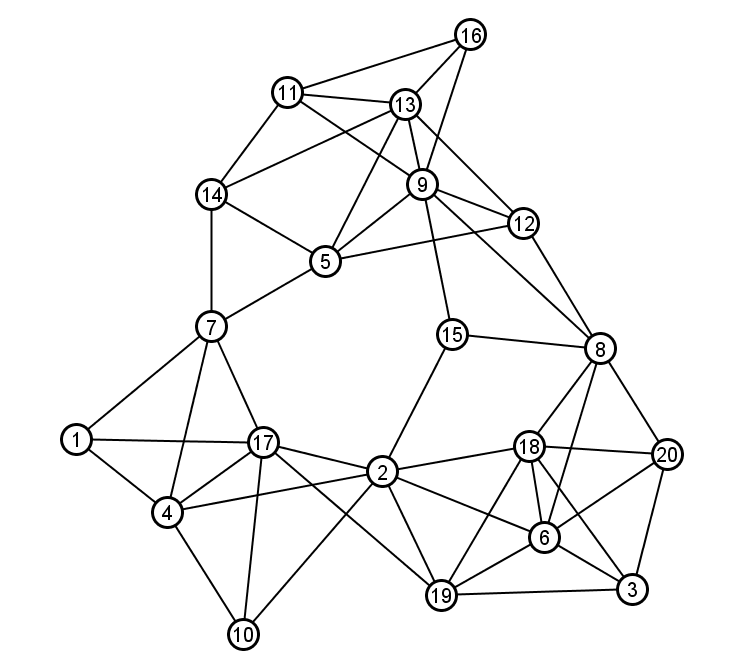The clustering coefficient of node 2:

Step 1: The neighborhood of node 2 is N(2) = {17, 4, 10, 19, 6, 18, 15}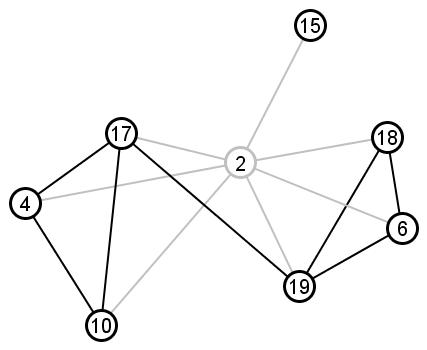Step 2: The subgraph induced by N(2) is indicated by the dark nodes and edges drawn at right. Node 2 is not an element of its own neighborhood, and the light gray edges are not part of the subgraph; those light gray elements are drawn just to provide context for the actual subgraph induced by N(2).

Step 3: Calculate the density of the induced subgraph using the usual formula, where our node set N(2) has 7 nodes and our edge set has 7 edges.

The maximum possible number of edges in the subgraph is

|N(2)|*(|N(2)|-1)/2 = 7 * 6/2 = 21

And the density of this subgraph is

= (actual # edges with both endpoints in N(2)) / (max possible # edges)

= 7/21 = 1/3

Thus the clustering coefficient of node 2 is 1/3.

The clustering coefficient of node 8:

Step 1: The neighborhood of node 8 is N(8) = {18, 6, 20, 12, 9, 15}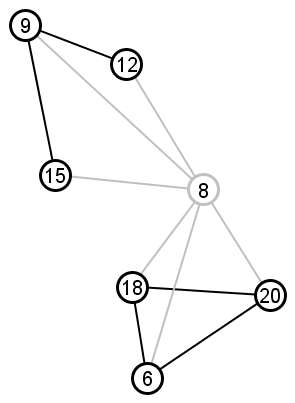Step 2: The induced subgraph is drawn at right (again the subgraph is indicated by dark nodes and edges and light gray elements are shown just for context)

Step 3: Calculate the density of the induced subgraph.

Number of nodes = 6

Number of edges = 5

Density = 5/(6*5/2) = 5/15 = 1/3

In this example, we again end up with a clustering coefficient of 1/3

The clustering coefficient of node 13:

Step 1: The neighborhood of node 13 is N(13) = {11, 14, 5, 9, 12, 16}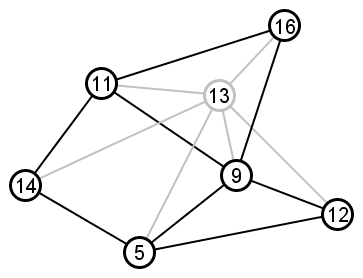Step 2: The induced subgraph is drawn at right (again the subgraph is indicated by dark nodes and edges and light gray elements are shown just for context)

Step 3: Calculate the density of the induced subgraph.

Number of nodes = 6

Number of edges = 8

Density = 8/(6*5/2) = 8/15

So the clustering coefficient of node 13 is 8/15.

# Network Structure

## Bipartite (Affiliation) Graphs

A bipartite graph (also called affiliation graph in Six Degrees) is a graph where the vertices can be partitioned into two independent sets.

A more intuitive way to think of a bipartite graph is as a graph whose nodes can each be colored red or blue such that there are no red-red edges and no blue-blue edges. The following graph, for example, is bipartite and so can be colored red and blue without creating red-red edges or blue-blue edges: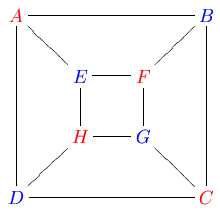Another way to think of a bipartite graph is by drawing the red nodes on one side of the picture and the blue nodes on the other side of the picture: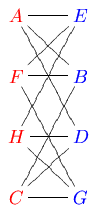Bipartite graphs are very commonly drawn in this way, with one subset of vertices on one side and the other subset on the opposite side. For the graph to be bipartite, edges may join vertices across columns but may never join vertices in the same column.

Bipartite graphs are useful for modelling matching problems. For example, which actors have worked on which movies? Which websites are bookmarked by which people? These are bipartite graphs.

Most graphs are non-bipartite. A graph is non-bipartite when there is:
1. No way to partition its vertices into two independent sets
2. No way to color its vertices red and blue without creating red-red or blue-blue edges
3. No way to draw its vertices in two columns so that edges only cross from column to column and never join vertices in the same column
Note that the above three condidtions are allsaying the same thing. For example, the following graph is non-bipartite:

Suppose we color the first node red; then we must color the second node blue. But then there is no way to color the third node red or blue without creating either a red-red edge or a blue-blue edge.

## Social Bookmarking and Bipartite Networks

One application of bipartite networks is the organization of user-created tags, such as the tags in del.icio.us. Together with the website URLs they are associated with, these tags form a bipartite network. For example, the yellow nodes below left are tags on del.icio.us, and the orange nodes below right are some of the URLs associated with these tags: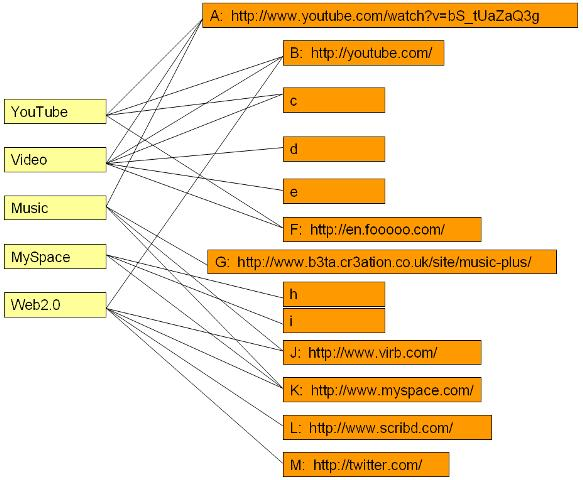## Structural Equivalence

Even a machine that doesn't understand the tag-words themselves can analyze how the tag-words relate to each other based on how others associate those tags with website URLs.

The simplest indicator of tag relatedness is the group interlock network, which is explained in Six Degrees. Unfortunately, the group interlock network is too crude for many purposes, because it provides only a yes/no indicator and does not indicate relative amount of relatedness.

A more useful measurement of tag (or node) relatedness is based on comparing network neighborhoods. Two nodes (or tags) with similar neighborhoods are closely related to each other; two nodes with completely different neighborhoods are not at all related, and there are many possible shades of grey in between.

In the example above, the neighborhoods of YouTube and Video have many nodes in common and so they are closely related. By contrast, the neighborhoods of MySpace and Video have no nodes in common and so they are not at all related (in the example--probably different case than real life).

We formalize this notion by defining the structural equivalence of two nodes x and y as

SE(x,y) = |N(x) ∩ N(y)| / |N(x) ∪ N(y)|

This equals 1 when two nodes have identical neighborhoods (i.e., two tags have identical website associations) and 0 when two nodes have mutually exclusive neighborhoods (i.e., two tags have not a single common website associated with them).

Examples:

The structural equivalence of YouTube and Video is

= |{A, B, C, F} ∩ {A, B, C, D, E, F}| / |{A, B, C, F} ∪ {A, B, C, D, E, F}|

= |{A, B, C, F}| / |{A, B, C, D, E, F}|

= 4 / 6

= 2/3

The structural equivalence of MySpace and Video is

|N(MySpace) ∩ N(Video)| / |N(MySpace) ∪ N(Video)|

=|{H, I, K} ∩ {A, B, C, D, E, F}| / |{H, I, K} ∪ {A, B, C, D, E, F}|

=|{ }| / |{A, B,C, D, E, F, H, I, K}|

= 0 / 9

= 0

## Trees

A tree is a graph in which any two vertices are connected by exactly one path.

Here are three different trees made from the same set of nodes:### Rooted (or Hierarchical) Trees

In computer science, we often use trees for hierarchical organization of data, in which case we use rooted trees.In the above tree, node 3 is specially designated as the root of the tree. Every other node in the tree is implicitly ranked by its distance from the root.

For any edge in a rooted tree, one end of the edge must be closer to the root than the other. We call the node closer to the root the parent of the other node, and we call the node farther from the root a child of its parent node. For example, nodes 9 and 4 are children of node 3. Similarly, nodes 10, 6, and 5 are all children of node 4. (And node 2 is the only child of node 9.) Any node in a rooted tree may have any number of children (including zero). A node with no children is called a leaf (examples leaf nodes are 1, 10, 7, and 5). Every node except the root node must have exactly one parent. For example, the parent of node 7 is node 6. Note that the above rooted tree is undirected, but each edge in the rooted tree has an implicit direction based on the parent-child relationship represented by that edge.

Any node of a tree can be a valid root of that tree. For example, if we decided that node 6 was actually the central anchor of the above network, we could re-root the exact same tree at 6 instead of 3:Check and see that the two graphs above have exactly the same sets of nodes and edges. What happens to parent-child relationships when a tree is re-rooted?

In terms of its graph theoretic properties, a rooted tree is equivalent to the traditional hierarchical organizational chart:The Division Officer at the top of the above hierarchy would probably argue that changing the root of the corresponding tree is a bad idea. However, if we are using trees to connect computers or concepts rather than people, we are less likely to encounter resistance when considering different alternatives for designating the root of a tree.

### Important properties of trees

A tree (rooted or not) may also be defined by the following equivalent conditions:
1. A connected graph with no cycles.
2. A connected graph with |E| = |V| - 1
Definition #1 above gives us an easy way to eyeball a graph and see if it is a tree. Does the graph consist of one connected component or does it fall apart into several connected components? A tree must be connected. Does the graph have any cycles (intuitively, a path that ends where it started)? A tree cannot have any cycles.

Which of the following three graphs are trees or not trees?Definition #2 above gives us a precise relationship between the number of nodes and edges in a tree, which can be very useful when designing algorithms that involve trees.

It is somewhat remarkable that these three conditions are completely equivalent:
1. G is a connected graph with no cycles.
2. G is a connected graph with |E| = |V| - 1.
3. G is a graph in which any two vertices are connected by exactly one path.
To understand better why these three are in fact equivalent, we will use mathematical induction.

### Mathematical Induction

When you write a computer program, how do you know it will work? A mathematical proof is a rigorous explanation of such a claim. (Example claim: "MapQuest finds the shortest path between any two addresses in the United States.") A proof starts with statements that are accepted as true (called axioms) and uses formal logical arguments to show that the desired claim is a necessary consequence of the accepted statements.

Mathematical induction (or simply induction) is one of the most important proof techniques in all of computer science. Induction is a systematic way of building from small example cases of a claim to show that the claim is true in general for all possible examples.

Every proof by induction has two parts:
1. Base case: Look at the smallest possible example case of the desired claim and show that the claim is true. The base case is often disarmingly trivial.
2. Inductive step: Here we get to assume the inductive hypothesis: that the claim holds for all example case of size i or less. Given that assumption, we must prove that the claim also holds for all example cases of size i+1.
That's it! Here's an example proof by induction. We will prove something we discussed in class weeks ago:

Suppose we want to prove that the maximum possible number of edges in an undirected graph with n nodes is n*(n-1)/2. Let's prove this by induction:
1. Base case: Consider a graph with one node. This graph can have no more than 0 edges. Checking our claimed formula when n=1, we verify 1*(1-1)/2 = 0.
2. Inductive step: The inductive hypothesis lets us assume that any graph with i nodes can have i*(i-1)/2 edges but no more. Now we have a graph with n=i+1 nodes. What is the maximum possible number of edges in this graph? We hope to show that this is (i+1)*((i+1)-1)/2 = (i+1)*i/2.
We take advantage of our inductive hypothesis by aribitrarily numbering the nodes of our graph 1, 2, 3, ..., i, i+1. Then we consider the first i nodes as one part of our graph and node i+1 as the other part of our graph:To count the maximum possible edges in our graph, we consider
1. The maximum possible number of edges in the red circle (nodes 1, 2, 3, ..., i)
2. Plus the maximum possible number of edges outside the red circle--those edges incident to node i+1
The inductive hypothesis tells us the maximum possible number of edges in the red circle is i*(i-1)/2.

The maximum number of edges incident to node i+1 is simply i (which would happen if node i+1 is adjacent to every other node in the graph).

Adding the above two terms gives us the maximum number of edges in a graph with n=i+1 nodes equal to
 i*(i-1) ------- + i 2 = i2 - i + 2i ----------- 2 = (i+1)*i -------- 2

Voila! Check for yourself that we have completed both the base case and the inductive step. We now have a complete proof by induction that the maximum number of edges in a graph with n nodes is n*(n-1)/2.

Now we return to our original goal, to understand better why these three conditions are equivalent:
1. G is a connected graph with no cycles.
2. G is a connected graph with |E| = |V| - 1.
3. G is a graph in which any two vertices are connected by exactly one path.
We will use induction to prove that a connected graph with no cycles has |E| = |V| - 1.
1. Base Case: Consider a graph with one node. A one-node graph is trivially connected and has no cycles. It cannot have any edges. We immediately get |E| = |V| - 1 = 0.
2. Inductive Step: Consider any connected graph with no cycles and no more than i nodes. The inductive hypothesis lets us assume that the number of edges is one less than the number of nodes for any such graph. Now consider a connected graph with no cycles and i+1 nodes. We aim to show that any such graph has exactly i edges, or that |E| = |V| - 1 = (i+1)-1 = i.
We take advantage of the inductive hypothesis by aribitrarily numbering the nodes of our graph 1, 2, 3, ..., i, i+1. Then we consider the first i nodes as one part of the graph and node i+1 as the other part of the graph.

Because the graph is connected, there is at least one edge from node i+1 to the rest of the graph. There may be more than one edge from node i+1 to the rest of the graph. Below we draw the case where there are three such edges:Here is the crux of the inductive step: If we remove node i+1 from the graph, then the rest of the graph breaks up into sub-trees. The number of sub-trees is exactly equal to the number of edges incident to node i+1.

To illustrate why this is so, we have colored the edges incident to node i+1 in the above example along with the sub-trees they connect to. Notice how each of the three "NO" scenarios violates one of our assumptions, either by creating a cycle (which either of the two red dashed edges would do) or by creating a disconnected graph (which the grey node would do).

Re-iterating: However many edges are incident to node i+1, then the nodes 1, 2, 3, ..., i break up into exactly that many sub-trees when we remove node i+1 and its edges.

Because each of the sub-trees has no more than i nodes, we can use the inductive hypothesis to tell us that each sub-tree has exactly one less edge than it has nodes.

In the above example, this means
 # edges in blue sub-tree = # edges in green sub-tree = # edges in yellow sub-tree = |Vblue| - 1 |Vgreen| - 1 |Vyellow| - 1

Although we don't know how many nodes are in each sub-tree, we do know the total number of nodes in all the sub-trees is i. So if we add the three equalities above, we get

 # edges in all sub-trees = i - 3
Now we add the three edges incident to node i+1 (three--as drawn in the example above) to get

 |E| = i - 3 + 3 = i

Three is a number we arbitrarily chose for the sake of this example--and notice how the 3's cancel each other out in the above sum. No matter whether there are 3, 2, 1, or whatever number of edges incident to node i+1, when we look at a connected graph with no cycles and i+1 nodes, we will end up with |E| = i, just as we aimed to prove. This completes our proof by induction that a connected graph with no cycles has |E| = |V| - 1.

## Minimum cost trees

Suppose your new office building is being wired for Internet. For the service to be worth much, your network must be connected (a path between every pair of computers); however, you probably don't want to install direct cables connecting every possible pair of computers.

Trees provide a useful starting point for any network service provider facing this sort of problem. This is because a tree connects a network with the minimum possible number of edges. (Think about why this is true.)

Simply finding any tree to connect a network isn't enough though. Not all computers (or nodes) are equally costly to join directly. Consider the network below:There are numbers next to each edge. Each number represents the cost (or weight) of the edge. In the above example, the weight of each edge corresponds to how far apart its endpoints are.

Suppose we want to choose a subset of edges to connect the above 7 nodes in a tree. Any tree connecting 7 nodes must have exactly 6 edges, so finding a "minimum edge tree" is meaningless. What we want are the 6 edges that form a tree for the least total cost.

We call this problem the minimum cost tree problem. It is often called the minimum spanning tree problem--"spanning" being a term applying to any subgraph that includes every possible node of the original graph.

The minimum cost tree problem turns out to one that is both remarkably important in real life and also easy to compute. Below is an algorithm that starts with a graph G = (V,E) and computes a set of edges T that form a tree (V,T) that has minimum possible total cost:
1. create a set T, initially equal to { }
2. create a set F, initially equal to E
3. if F = { } then go to step 7
4. remove an edge {x,y} with minimum cost from F
5. if x and y are already connected by a path of edges in T, then discard edge {x,y}; otherwise add edge {x,y} to T
6. go to step 3
7. done--the minimum cost tree is (V,T)
The above algorithm is called Kruskal's algorithm.

### Example

We will apply Kruskal's algorithm to the graph above. Recall that set F initially includes every edge. Set T is initially empty. As we add edges to T in the example below, we color those edges green.This is our original graph. The numbers near the arcs indicate their weight. None of the arcs are highlighted.{A,D} and {C,E} are the shortest arcs, with length 5, and {A,D} has been arbitrarily chosen, so it is highlighted.However, {C,E} is now the shortest arc that does not form a cycle, with length 5, so it is highlighted as the second arc.The next arc, {D,F} with length 6, is highlighted using much the same method.The next-shortest arcs are {A,B} and {B,E}, both with length 7. {A,B} is chosen arbitrarily, and is highlighted. The arc {B,D} has been highlighted in red, because it would form a cycle (A,B,D,A) if it were chosen.The process continutes to highlight the next-smallest arc, {B,E} with length 7. Many more arcs are highlighted in red at this stage: {B,C} because it would form the cycle (B,C,E,B) {D,E} because it would form the cycle (D,E,B,A,D) {F,E} because it would form cycle (F,E,B,A,D,F).Finally, the process finishes with the arc {E,G} of length 9, and the minimum spanning tree is found.

## PageRank and Centrality

Google's search engine relies heavily on network mathematics--and PageRank in particular--to rank websites and automatically calculate which ones are the "best" answer to a query.

### Centrality

The core network mathematical concept in Google rankings is the notion of centrality. This is an intuitive notion that is surprisingly hard to pin down mathematically. For example, which node is the most central in the following graph?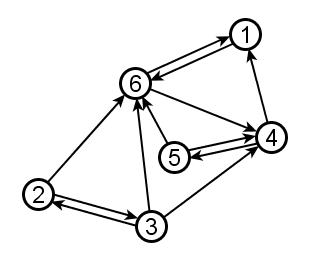There is no one right answer to the question, because the question is too vague. There are many different ways to define centrality more precisely. Here are three important ones, all of which rely critically on the direction of links (something we have been glossing over until now):

• Outdegree Centrality: Recall the difference between indegree and outdegree (see Degree). "Outdegree centrality" defines centrality as a measure of how many links point out of a node. A webpage that has many clickable links on the screen has high outdegree centrality. A webpage with very few clickable links has low outdegree centrality. In the above graph, node 3 has the highest outdegree centrality; it is the only node with 3 outgoing links.
• Indegree Centrality defines centrality as a measure of how many links point into a node. A webpage that is linked to by many other webpages has high indegree centrality. A webpage that is not linked to by many other webpages has low indegree centrality. Node 6 above has the highest indegree centrality; it is the only node with 4 incoming links. Indegree centrality corresponds roughly to the popularity of a website.
• PageRank is a more sophisticated version of indegree centrality. The key refinement is that not every incoming link counts equally in computing PageRank--links from more popular neighbors count more than links from less popular neighbors. For example, notice that nodes 2, 3, and 5 all have equal indegree centrality (which is 1). However, node 5 is recommended by node 4, which is a very popular node. Nodes 2 and 3 are not popular and only receive recommendations from each other. So node 5 has significantly higher PageRank than nodes 2 and 3.

The PageRank formula is described and illustrated with examples and an interactive PageRank calculator at http://www.webworkshop.net/pagerank.html.

WebWorkshop writes the PageRank formula in a manner that is compact but subtle. We restate the formula here and then introduce an easier, simplified forumulation. We begin by defining a damping factor d to be a fixed coefficient at least 0 but no more than 1. (A typical value is d=0.85.) Then for any graph, we define the PageRank of each of its nodes as follows:

 For any node x, let {y1, y2, ..., yn} denote the set of all nodes with directed links to x, Then PageRank(x) = (1-d) + d*(PageRank(y1)/Outdegree(y1) +PageRank(y2)/Outdegree(y2) ... + PageRank(yn)/Outdegree(yn))

Computing PageRank from the above definition presents both major and minor difficulties:

• The PageRank formula is defined in terms of itself, presenting us with a "Catch-22" situation. We will manage this Catch-22 by starting with a very crude estimate of PageRank, and then running repeated iterations of calculations, which produce ever-more-accurate results by building on results of previous iterations.
• Even once we accept the iterative approach to PageRank, the calculation still involves multiplying by a damping coefficient and dividing by node-outdegree, resulting in much messier fractions than we like to present in this course.

Here we present Compounded Indegree Centrality as a simple way to estimate PageRank. It is equivalent to the PageRank formula referenced above with damping coefficient d=1 and with no dividing by node outdegrees. Compounded indegree centrality will proceed iteratively, by first calculating InDegree1, then InDegree2 based on InDegree1, and so forth.

Using the same 6-node graph as above, we start by defining the InNeighborhood of a node x to be all nodes that have directed edges into x. Then the InDegree1 of a node is simply the cardinality of its InNeighborhood--just another name for indegree.Node InNeighborhood InDegree1 1 {4,6} 2 2 {3} 1 3 {2} 1 4 {3,5,6} 3 5 {4} 1 6 {1,2,3,5} 4

InDegree1 is a very local measure of node centrality. We can measure a slightly more system-wide sense of node centrality by defining InDegree2 as follows: For any node x, InDegree2 of x is the sum of the InDegree1's of all nodes in the InNeighborhood of x.

 Node InNeighborhood InDegree1 InDegree2 1 {4,6} 2 7 = 3+4 2 {3} 1 1 = 1 3 {2} 1 1 = 1 4 {3,5,6} 3 6 = 1+1+4 5 {4} 1 3 = 3 6 {1,2,3,5} 4 5 = 2+1+1+1

We can then repeat the above process to calculate InDegree3 based on InDegree2, then InDegree4 based on InDegree3, etc. Below is a table of five successive calculations of InDegree, based on the same graph as above:

 Node InNeighborhood InDegree1 InDegree2 InDegree3 InDegree4 InDegree5 1 {4,6} 2 7 11 21 38 2 {3} 1 1 1 1 1 3 {2} 1 1 1 1 1 4 {3,5,6} 3 6 9 19 29 5 {4} 1 3 6 9 19 6 {1,2,3,5} 4 5 12 19 32

Below we redraw the same table, highlighting exactly how the row for node 4 is calculated. Because the InNeighborhood of node 4 is {3,5,6}, in each iteration the compounded indegree of node 4 takes the values of nodes 3, 5, and 6 from the previous column.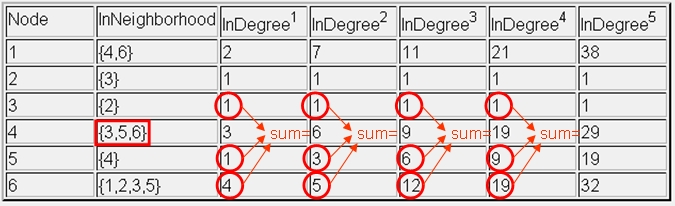Notice also that the rankings of relative node centrality change from column to column in the above table. Sometimes node 6 is most central; sometimes node 1 is most central. After "enough" iterations, the rank order of relative node centrality will stabilize.